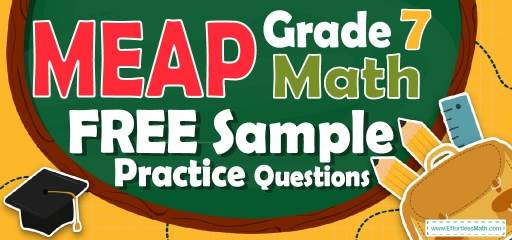# 7th Grade MEAP Math FREE Sample Practice QuestionsIn order to succeed in the 7th Grade MEAP Math exam, students must not only have sufficient study but also should be familiar with the various questions of the exam. It is important for 7th Grade students to know that time management is very important both when studying and practicing. To strengthen 7th Grade students’ test skills, it is best to use 7th Grade MEAP Math sample practice questions. We have provided examples of the best 7th Grade MEAP Math Sample Practice Questions in this article. We hope that by using these questions, students will be able to improve their skills to pass the 7th Grade MEAP Math test.

Start preparing your student for the 2022 MEAP Math test with our free sample practice questions. Also, make sure to follow some of the related links at the bottom of this post to get a better idea of what kind of mathematics questions students need to practice.

## 10 Sample 7th Grade MEAP Math Practice Questions

1- A chemical solution contains $$4\%$$ alcohol. If there is 24 ml of alcohol, what is the volume of the solution?

A. 240 ml

B. 480 ml

C. 600 ml

D. 1200 ml

2- The price of a laptop is decreased by $$10\%$$ to $360. What is its original price? A. 320 B. 380 C. 400 D. 450 3- What is the median of these numbers? $$4, 9, 13, 8, 15, 18, 5$$ A. 8 B. 9 C. 13 D. 15 4- Three times the price of a laptop is equal to five times the price of a computer. If the price of the laptop is$200 more than a computer, what is the price of the computer?

A. 300

B. 500

C. 800

D. 1500

5- What is the perimeter of a square that has an area of 595.36 feet?

6- Jason is 9 miles ahead of Joe running at 5.5 miles per hour and Joe is running at the speed of 7 miles per hour. How long does it take Joe to catch Jason?

A. 3 hours

B. 4 hours

C. 6 hours

D. 8 hours

7- 55 students took an exam and 11 of them failed. What percent of the students passed the exam?

A. $$20 \%$$

B. $$40 \%$$

C. $$60 \%$$

D. $$80 \%$$

8- Jason needs an $$75\%$$ average in his writing class to pass. On his first 4 exams, he earned scores of $$68\%, 72\%, 85\%,$$ and $$90\%$$. What is the minimum score Jason can earn on his fifth and final test to pass?

9- A bank is offering $$3.5\%$$ simple interest on a savings account. If you deposit $12,000, how much interest will you earn in two years? A.$420

B. $840 C.$4200

D. $8400 10- 5 less than twice a positive integer is 83. What is the integer? A. 39 B. 41 C. 42 D. 44 ## Best 7th Grade MEAP Math Workbook Resource for 2022 ## Answers: 1- C $$4\%$$ of the volume of the solution is alcohol. Let $$x$$ be the volume of the solution. Then: $$4\% \space$$ of $$\space x = 24 \space$$ ml $$⇒ 0.04 x = 24 ⇒ x = 24 ÷ 0.04 = 600$$ 2- C Let $$x$$ be the original price. If the price of a laptop is decreased by $$10\%$$ to$360, then:
$$90 \%$$of $$x=360 ⇒ 0.90x=360 ⇒ x=360÷0.90=400$$

3- B
Write the numbers in order:
$$4, 5, 8, 9, 13, 15, 18$$
Since we have 7 numbers (7 is odd), then the median is the number in the middle, which is 9.

4- A
Let L be the price of laptop and C be the price of computer.
$$3$$(L) $$=5$$(C) $$\space$$ and $$\space$$ L $$= 200 +$$ C
Therefore, $$3(200 +$$ C$$)=5$$C $$⇒ 600 + 3$$C $$= 5$$C $$⇒$$ C$$=300$$

5- 97.6
Use the area of square formula.
$$S = a^2 ⇒ 595.36 = a^2 ⇒ a = 24.4$$
Use the perimeter of square formula.
$$P = 4a ⇒ P=4(24.4) ⇒ P = 97.6$$

6- C
The distance between Jason and Joe is 9 miles. Jason running at 5.5 miles per hour and Joe is running at the speed of 7 miles per hour. Therefore, every hour the distance is 1.5 miles less.
$$9 ÷ 1.5 = 6$$

7- D
The failing rate is 11 out of $$55 = \frac{11}{55}$$.
Change the fraction to percent:
$$\frac{11}{55} ×100\%=20\%$$
20 percent of students failed. Therefore, 80 percent of students passed the exam.

8- 60
Jason needs an $$75\%$$ average to pass for five exams. Therefore, the sum of 5 exams must be at lease $$5 × 75 = 375$$
The sum of 4 exams is:
$$68 + 72 + 85 + 90 = 315$$
The minimum score Jason can earn on his fifth and final test to pass is:
$$375 – 315 = 60$$

9- B
Use simple interest formula:
I=prt
(I = interest, p = principal, r = rate, t = time)
I$$=(12000)(0.035)(2)=840$$

10- D
Let $$x$$ be the integer. Then:
$$2x – 5 = 83$$
Add 5 both sides: $$2x = 88$$
Divide both sides by $$2: x = 44$$

## The Best Books to Ace 7th Grade MEAP Math Test

### What people say about "7th Grade MEAP Math FREE Sample Practice Questions - Effortless Math: We Help Students Learn to LOVE Mathematics"?

No one replied yet.

X
27% OFF

Limited time only!

Save Over 27%

SAVE $5 It was$18.99 now it is \$13.99